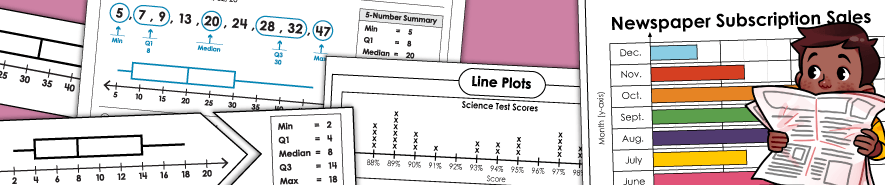# Graphing WorksheetsBar Graph Worksheets

Read, create, and interpret bar graphs with these worksheets.

Box Plots (Box-and-Whisker Plots)

Create box plots on a number line using the values for Q1, median, Q3, minimum, and maximum.
(Approx grade levels: 6, 7, 8)

Line Graph Worksheets

Read and create line graphs with these worksheets.

Line Plot (Dot Plot) Worksheets

Learn how to plot frequency of data on a number plot number line. These are sometimes called dot plots.

Ordered Pair Worksheets

Discover how to plot ordered pairs on coordinate grids.

Ordered Pair Graph Art

Plot the points of the grids and connect with lines to make pictures.

Pictograph Worksheets

Interpret data on these picture-symbol graphs.

Pie Graph Worksheets

Analyze circle graphs, also known as pie graphs, which show fractions and percentages of a whole.

Math Worksheets

This takes you to our full index of math worksheets.